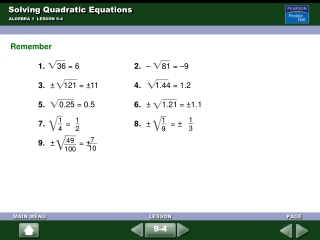DownloadDownload Presentation# Solving Quadratic Equations

Télécharger la présentation## Solving Quadratic Equations

- - - - - - - - - - - - - - - - - - - - - - - - - - - E N D - - - - - - - - - - - - - - - - - - - - - - - - - - -
##### Presentation Transcript

1. 1 4 1 9 49 100 7 10 Solving Quadratic Equations ALGEBRA 1 LESSON 9-4 Remember 1. 36 = 6 2. – 81 = –9 3. ± 121 = ±11 4. 1.44 = 1.2 5. 0.25 = 0.5 6. ± 1.21 = ±1.1 7. = 8. ± = ± 9. ± = ± 1 3 1 2 9-4

2. Graph y = 2x2 Graph y = 2x2 + 2 Graph y = 2x2 – 2 Solving Quadratic Equations ALGEBRA 1 LESSON 9-4 Solve each equation by graphing the related function. a. 2x2 = 0 b. 2x2 + 2 = 0 c. 2x2 – 2 = 0 There is one solution, x = 0. There is no solution. There are two solutions, x = ±1. 9-4

3. 3x2 – 75 + 75 = 0 + 75Add 75 to each side. 3x2 = 75 x = ± 25 Find the square roots. Solving Quadratic Equations ALGEBRA 1 LESSON 9-4 Solve 3x2 – 75 = 0. x2 = 25 Divide each side by 3. x = ± 5 Simplify. 9-4

4. S = 4 r2 315 = 4 r2 Substitute 315 for S. = r2 Put in calculator ready form. 315 (4) 315 (4) Find the principle square root. = r2 Use a calculator. 5.00668588 r Solving Quadratic Equations ALGEBRA 1 LESSON 9-4 A museum is planning an exhibit that will contain a large globe. The surface area of the globe will be 315 ft2. Find the radius of the sphere producing this surface area. Use the equation S = 4 r2, where S is the surface area and r is the radius. The radius of the sphere is about 5 ft. 9-4

5. 3 7 ± Solving Quadratic Equations ALGEBRA 1 LESSON 9-4 1. Solve each equation by finding square roots. If the equation has no solution, write no solution. a. 2x2 – 8 = 0 b.x2 + 2 = –2 2. Solve each equation by finding square roots. a.m2 – 25 = 0 b. 49q2 = 9 3. Find the speed of a 4-kg bowling ball with a kinetic energy of 160 joules. Use the equation E = ms2, where m is the object’s mass in kg, E is its kinetic energy, and s is the speed in meters per second. ±2 no solution ±5 1 2 about 8.94 m/s 9-4